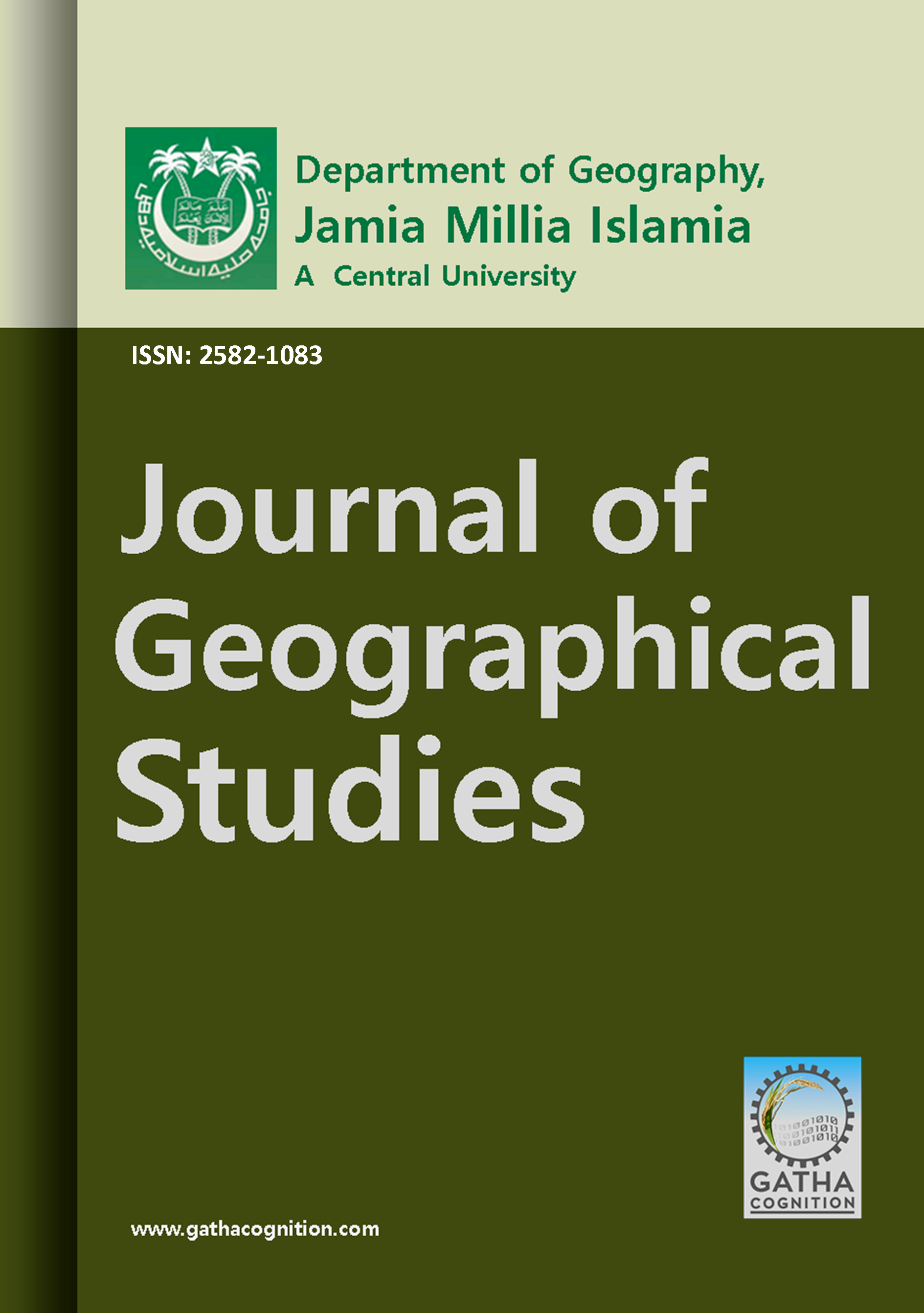1 (2017), 1, 3-10

### Journal of Geographical Studies

2582-1083

#### Modeling Surface Soil Moisture from Microwave Remote Sensing Data in Solani River Catchment Uttarakhand India

1.Department of Civil Engineering, Z. H. College of Engineering and Technology, Aligarh Muslim University (AMU), Aligarh U.P. (India).

Dr.Saif Said*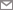*.Department of Civil Engineering, Z. H. College of Engineering and Technology, Aligarh Muslim University (AMU), Aligarh U.P. (India).

Professor.Masood Ahsan Siddiqui 11.Department of Geography, Jamia Millia Islamia – A Central University, New Delhi-110025 (India).

07-07-2017
03-06-2017
03-07-2017
04-07-2017

#### Highlights

1. ERS-2 SAR image has been utilized for estimating surface soil moisture by incorporating the effect of topography, vegetation and surface roughness over three land cover types namely; sugarcane, wheat and barren land through Multiple Linear Regression (MLR) approach.
2. Five independent variables considered for MLR analysis include backscatter coefficient, local incidence angle, surface roughness height, Leaf Area Index and Plant Water Content, respectively.
3. Higher accuracy was observed for barren land as compared to the other two land cover types (i.e. sugarcane and wheat).
4. The present study therefore reveals the effectiveness of MLR approach in the retrieval of surface soil moisture while utilising fewer number of independent variables through microwave remote sensing data.

#### Abstract

The spatiotemporal variation of surface soil moisture is a key subject variable need to be assessed accurately since it plays a crucial role in partitioning of rainfall into runoff and infiltration. Active microwave remote sensing has offered prominent potential towards accurate estimation of surface soil moisture. Present study utilizes ERS-2 SAR image for estimating surface soil moisture by incorporating the effect of topography, vegetation and surface roughness over three land cover types namely; sugarcane, wheat and barren land through Multiple Linear Regression (MLR) approach. Five independent variables considered for MLR analysis include backscatter coefficient ($σ^0$ ), local incidence angle ($α_i$), surface roughness height ($h_s$), Leaf Area Index (LAI) and Plant Water Content (PWC), respectively. Results indicate retrieval of soil moisture within ± 20% accuracy for all the three land cover types with higher accuracy for barren land (i.e. R2 ~ 0.78 and RMSE = 1.31) as compared to the other two land cover types (i.e. sugarcane; R2 ~ 0.67 and RMSE = 3.60 and wheat; R2 ~ 0.72 and RMSE = 1.94). The present study reveals the effectiveness of MLR approach in the retrieval of surface soil moisture using fewer numbers of variables.

#### Keywords

Surface Soil Moisture , Backscatter Coefficient , Leaf Area Index (LAI) , Multiple Linear Regression (MLR) , Solani River , ERS-2 SAR

#### 1 . INTRODUCTION

Soil moisture is the amount of water held in the soil pores within the top 5 to 10 cm layer from the earth’s surface and constitutes to a mere 0.005% of the global water. Despite its small amount, soil moisture plays a crucial role in controlling and regulating the interaction between the atmosphere and the land surface as well as partitioning of precipitation into runoff and ground water storage. Soil moisture can directly be measured at selected locations by using conventional methods but may not be applied where its estimation is required at regional and global scales. Also, quantification of soil moisture in terms of spatial and temporal distribution enhances its interpretation required in numerous studies including hydrology, meteorology, agriculture and irrigation scheduling (Dobson and Ulaby, 1986).

Microwave region of electromagnetic spectrum has the potential for estimating soil moisture at spatial and temporal scales (Ulaby et al., 1986; Shepard, 1998; Bindlish and Barros, 2000; Sahebi et al., 2003, Haider et al., 2004; Song et al., 2008; Gharechelou et al., 2015). For more than two decades, a range of models and approaches have been developed for soil moisture estimation from microwave remote sensing (Ulaby et al., 1982; Fung and Chang 1992; Prévot et al., 1993; Baghdadi, 2006). The radar backscatter coefficient ‘s0’ a function of digital number (DN) of Synthetic Aperture Radar (SAR) has been found to be related with volumetric soil moisture. However, it is highly influenced by dielectric constant and surface roughness conditions of the barren land and vegetative cover in agricultural fields. Therefore, effect of topography, vegetation density and variation in small scale surface roughness on s0 is required to be taken into account for accurately estimating the soil moisture. Existing models

for correlating soil moisture with s0 can be grouped into three categories; empirical, theoretical and semi-empirical models wherein only approximate solutions can be derived pertaining to the validity of the underlying assumptions. For instance, empirical models (i.e. Dubois, 1995 and Oh, 1992) and theoretical models such as Physics Optical Model (POM), Geometric Optics Model (GOM), Small Perturbation Model (SPM) and Integral Equation Model (IEM) may be applicable to surfaces with specific roughness characteristics with limited validity ranges may not represent prevalent soil moisture conditions. Semi-empirical models such as Radiative Transfer Model (RTM), and Wave Model (WAM) (Ulaby, et al., 1986) are applicable for a wide range of surfaces but requires extensive in-situ surface measurements and complex mathematical equations exhibiting high non-linearity.

To circumvent these limitations, non-parametric approaches namely; Artificial Neural Network (ANN), Genetic Algorithm (GA), fuzzy logic as well as Multiple Linear Regression (MLR) have been employed by some researchers to estimate soil moisture from microwave remote sensing data. ANN for instance, offers a simple solution to the problems possessing the nonlinear relationship between the dependent and independent variables (Bindlish and Barros, 2000; Sahebi et al., 2003; Said et al., 2008). Few studies (Jackson et al., 1996; Mattikalli et al., 1998; Zhao and Li, 2013 and Li et al., 2014) have focused on multiple linear regression approach establishing correlations between observed remotely sensed observations namely digital number or backscatter coefficient and surface soil moisture. The present study however, attempts to relate fewer numbers of variables namely backscatter coefficient, local incidence angle, surface roughness, leaf area index and plant water content bearing significant impact over soil moisture retrieval through multiple linear regression approach.

#### 2 . STUDY AREA AND DATA SETS

The catchment area of river Solani (a tributary of river Ganges) around Roorkee town (between, 77.68°E, 30.51°N and 77.47°E, 29.60°N), India, has been adopted as the study area (Figure 1(a)). The area is relatively flat with an average slope of 5° and a maximum of 9°, constitutes barren land in addition to mixed vegetation with major agricultural crop being sugarcane, wheat,  rice and fodder crop. The beginning of the autumn season has fairly good amount of rainfall and average humidity of 45% to 50%. Crops were at their mature stage at the time of field data collection which led to the variation in both surface roughness and moisture content within the fields and the area had effectively no rainfall at that time.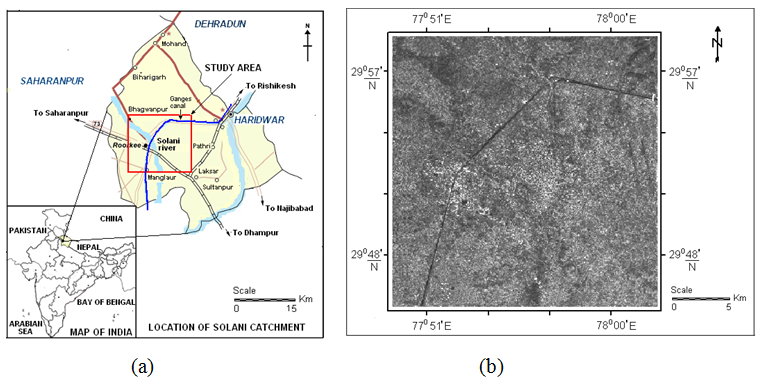#### Figure 1. (a) Location of the study area (Rectangular box shows the Solani river catchment under study) (b) Georeferenced ERS-2 SAR image of study area acquired on 29th March 2004

The usefulness of the remote sensing data in terms of the retrieved soil moisture can be established by comparing the estimated values of the soil moisture with concurrently measured data in-situ. ERS-2 SAR C- band image (5.3GHz frequency and VV polarization) at spatial resolution of 12.5 m acquired on 29th March 2004 was procured from National Remote Sensing Center (NRSC), Hyderabad (Figure 1(b)). RMS surface roughness height ‘ $h_s$ ’ vegetation parameters namely; plant and leaf samples for estimating LAI and PWC as well as soil samples at selected locations were collected

concurrent to the date of satellite overpass. A total of 135 soil samples (45 each for the three selected land cover classes, namely; sugarcane, wheat and barren land) were collected from within the entire selected study area. Global Positioning System (GPS) survey was conducted to obtain the accurate coordinates of each sampling location that can be tagged in the image so as to extract the corresponding pixel values or digital numbers.

Soil surface roughness can be measured through a number of methods proposed by researchers but these are cumbersome and involve lengthy processes of measurement. Therefore, a mechanical device named here as ‘surface roughness profiler’ was designed and fabricated in the laboratory to measure the rms surface roughness heights ‘ $h_s$ ’ (Figure 2).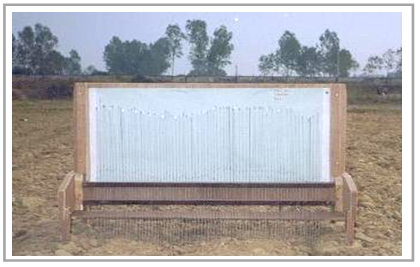#### Figure 2. Surface roughness profiler for measuring the rms soil surface roughness in fields

The profiler has a base length of 85 cm with 70 vertical needles of height 70 cm each placed at an equal interval of 1 cm. The profiler was placed at 3 to 4 locations around each sampling point in the field to collect the data pertaining to the surface roughness. The measurements were taken and averaged for that location. The rms heights ( $h_s$ ) of the surface were computed as (Bennet and Mattson, 1989):

$h_s = \sqrt{{\frac{1}{n} \sum _{x=1}^{n-1} \left[ S \left( x \right) -\overline{S} \left( x \right) \right] ^{2}}}$                                          (1)

where,  $s(x)$  is the surface height at a point $x$  in the surface profile, $\overline{S} \left( x \right)$  is the average height of the surface profile and $n$  is the total number of points (vertical rods)  along the abscissa.

#### 3 . METHODOLOGY

Following general methodology has been adopted in the present study for estimating soil moisture from active microwave remote sensing data.

#### 3.1  Geo-referencing of ERS-2 SAR Image

Acquired C-band microwave imagery was geo-referenced using eight ground control points, 1st order polynomial transformation and nearest neighbour re-sampling method.

#### 3.2  Estimation of Backscatter Coefficient $\sigma ^{0}$  from Digital Number (DN)

Speckle noise in the SAR image was smoothed using Lee-sigma filter in Erdas Imagine 9.2. $\sigma ^{0}$  and local incidence angle $\alpha _{i}$  were computed using an in-house program (in MATLAB 7.1) based on the algorithm proposed by European Space Agency (ESA) guidelines. The $\sigma ^{0}$  as a function of DN and $\alpha _{i}$  is given by;

$\sigma ^{0}= \left[ \frac{1}{N} \cdot \sum _{ij=1}^{ij=N}DN_{ij}^{2} \right] \cdot \frac{1}{K} \cdot \frac{sin \alpha _{i}}{sin \alpha _{ref}}$              (2)

where, $N$  is the number of pixels within the area of interest (i.e., the distributed target). $DN_{ij}$  is the digital number corresponding to the pixel at location ( $i, j$ ). $\alpha _{i}$ and $\alpha _{ref}$ are the local and average or midrange incidence angles, respectively. The value of $\alpha _{ref}$  for SAR sensor and $K$  is the calibration constant. The values of $\alpha _{ref}$ and $K$ , as obtained from the sensor’s ephemeris record are 23˚ and 889201.00, respectively.

The local incidence angle $\alpha _{i}$  for a pixel at range location $i$  may be computed as:

$cos \alpha _{i}=\frac{ \left( R_{T}+H \right) ^{2}-R_{i}^{2}-R_{T}^{2}}{2R_{i}R_{T}}$                                   (3)

where, $R_T$  is the radius of the Earth at the first position of satellite, $H$  is the altitude of ERS-2 satellite and $R_i$  is the slant range to a pixel at location $i$ .

#### 3.3  Crop Parameters

LAI is defined as the one-sided green leaf area per unit ground area and characterizes the density of vegetation in accordance to the size of the leaf that actively participates in weakening of the backscatter coefficient over vegetation (Paris, 1986). LAI can be computed using the following relation,

$LAI=~N_{a}\times A_{1}$                                             (4)

where, $N_a$  is the areal density of the scattering elements which was obtained from field measurements, $A_1$  is the one sided area of the leaf determined by on-screen digitisation of leaves on their scanned photographs.

Plant Water Content (PWC) is defined as the total amount of water (or moisture) present in the samples of plant leaves and stem. Since, dielectric properties of the vegetation are governed by PWC, plays a dominant part in attenuation processes of the backscatter coefficient (Attema and Ulaby, 1978). PWC can be computed from:

$PWC=\frac{W_{f}-W_{d}}{W_{d}}$                                               (5)

where, $W_f$  and $W_d$  are the freshly plucked and oven-dried weights of plant samples collected in the field.

#### 3.4  Volumetric Measurement of Soil Moisture

The standard method of measuring the volumetric soil moisture content of a soil sample is the gravimetric method also referred as the thermo-gravimetric method, which consists of oven drying the soil samples at 105°C and relating the change in mass to the volume of the sample. The computation of soil moisture is based on the following equation;

$m_{v}=\frac{W_{w}-W_{d}}{W_{d}} \times \frac{ \rho _{b}}{ \rho _{w}} \times 100$                                 (6)

where $m_v$  is the soil moisture, $W_w$  and $W_d$  are the weights of wet and dry soil, $\rho _{b}$  and $\rho _{w}$  are the bulk densities of soil and water.

#### 3.5  Multiple Linear Regression (MLR)

Multiple linear regression technique attempts to model the relationship between one or more explanatory variables and a response variable, which can be described by fitting a linear or a non-linear equation to the observed data. More precisely, multiple regression analysis helps us to predict the value of Y for given values of X1, X2, …, Xk. Multiple regressions can establish a set of independent variables having a fraction of the variance in a dependent variable at a significant level.

#### 4 . RESULTS AND DISCUSSION

Multiple linear regression analysis was performed on the data set collected through field visit conducted on the day of satellite pass. Forty five soil samples were collected from each of the three land cover types namely; sugarcane, wheat and barren land. Volumetric soil moisture was computed by oven drying the soil samples at 105° C and relating the change in mass to the volume of the sample. Small cylindrical tin cans of approximately 3.5 cm diameter and 6 cm height were used to collect soil samples from random locations within the study area. The present analysis utilizes 80% (~ 35 data samples) of the data to perform regression and the rest (~ 10 data samples) were used for validation purpose. Coordinates recorded through GPS corresponding to each sampling location were precisely tagged on the microwave image for the purpose of extraction of DN values illustrated in Table 1. Corresponding sensor, soil and plant related parameters were computed and measured and their statistical analysis is shown in Table 2. A total of five independent variables including   $\sigma ^{0}$ , LAI, PWC, $\alpha _{i}$  and $h_s$ were utilized to model volumetric soil moisture for sugarcane and wheat crops. However, only three variables namely; $\sigma ^{0}$ , $\alpha _{i}$  and $h_s$  were utilized for the case of barren land.

#### Table 1. Digital numbers extracted from ERS-2 SAR image

 Sr. No. Land cover classes Total no. of in-situ samples Digital Number Minimum Maximum 1 Sugarcane 45 411.73 458.27 2 Wheat 45 360.07 404.84 3 Barren Land 45 220.03 253.86

#### Table 2. Statistical data used for modeling soil moisture

 Sr. No. Land cover class Statistics Backscatter coefficient $\sigma ^{0}$  (dB) LAI PWC Local incidence angle $\alpha _{i}$  (Degrees) rms roughness   $h_s$  (cm) Volumetric soil moisture $m_v$  (%) 1 Sugarcane Mean -15.7863 4.907 0.8163 22.3609 1.295 14.37 S.E.M. 0.0929 0.0333 0.0041 0.0740 0.0022 1.08 S.D. 0.5494 0.1971 0.0244 0.4377 0.0129 6.38 Variance 0.3019 0.0388 0.0006 0.1916 0.0002 40.65 2 Wheat Mean -18.4903 3.311 0.6249 22.1437 1.358 11.15 S.E.M. 0.1232 0.0429 0.0011 0.0740 0.0043 0.63 S.D. 0.7287 0.2540 0.0066 0.4376 0.0254 3.72 Variance 0.5310 0.0645 0.0000 0.1915 0.0006 13.81 3 Barren Land Mean -28.06771 - - 22.4809 1.228 9.23 S.E.M. 0.10818 - - 0.1007 0.0034 0.49 S.D. 0.64002 - - 0.5955 0.0198 2.89 Variance 0.40962 - - 0.3546 0.0004 8.38

S.E.M. = Standard Error of Measurement; S.D. = Standard Deviation.

Table 3 illustrates the results obtained from MLR analysis in terms of regression coefficients, R2 and RMSE for calibration and validation data sets. Scatter plots between observed soil moisture and calculated soil moisture corresponding to the three classes are shown in Figure 3. Of the three land cover types, barren land accounted for the highest R2 (i.e. ~ 0.78 and 0.71) values for calibration as well as validation data sets. High R2 values for barren land can be attributed to the fact that in the absence of plants, only soil surface roughness contributes towards the attenuation in terms of scattering of radar backscatter that ultimately results in more accurate estimation of soil moisture.

#### Table 3. MLR Coefficients, R2 and RMSE values for Calibration and Validation of Data

 Sr. No. Land cover classes Parameter distribution Regression coefficients R2 (Calibration) RMSE R2 (Validation) RMSE 1 Sugarcane X1 = $\sigma ^{0}$ b0 = -27.2716 0.67 3.60 0.64 3.66 X2 = LAI b1 = 7.76015 X3 = PWC b2 = 2.60094 X4 = Angle b3 = 26.7792 X5 = $h_s$ b4 = 1.41067 b5 = 75.6829 2 Wheat X1 = $\sigma ^{0}$ b0 = -20.7238 0.72 1.94 0.66 1.00 X2 = LAI b1 = 1.81781 X3 = PWC b2 = 8.45773 X4 = Angle b3 = 53.6324 X5 = $h_s$ b4 = 0.36631 b5 = -3.0447 3 Barren Land X1 = $\sigma ^{0}$ b0 = -47.3752 0.78 1.31 0.71 2.04 X2 = Angle b1 = 1.15972 X3 = $h_s$ b2 = 2.80663 b3 = 21.2239

Multiple Regression Equation $Y=b_{0}+b_{1} \times X_{1}+b_{2} \times X_{3}+b_{3} \times X_{3}………$

Where $Y$  = Volumetric soil moisture

Data distribution: 80% correlation and 20% validation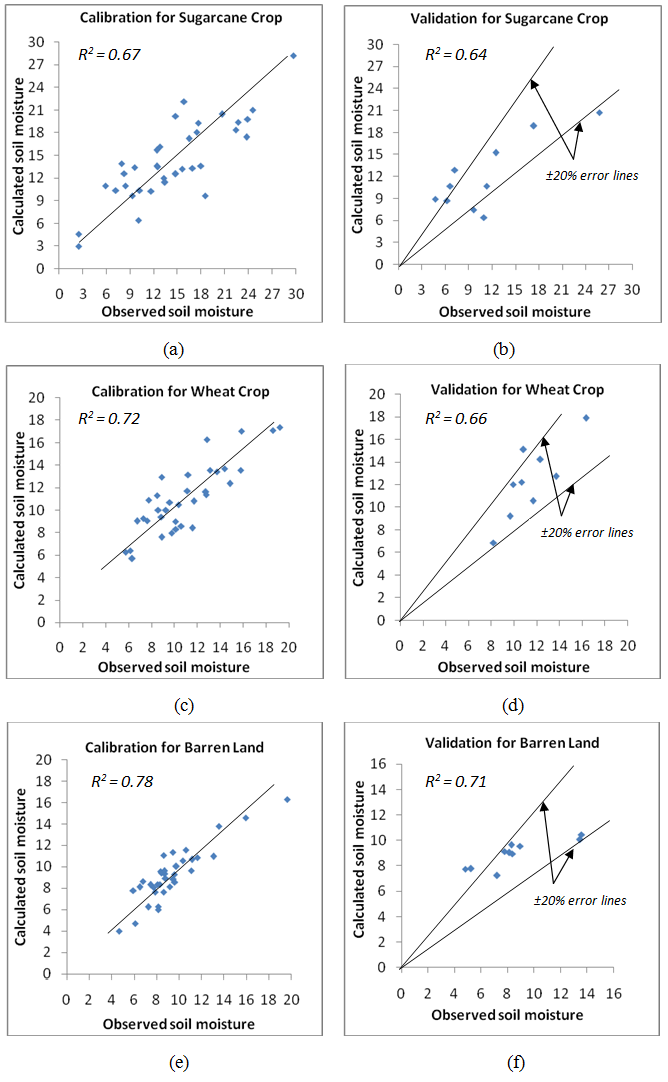#### Figure 3. Scatter plots between observed and calculated soil moisture values

Furthermore, sugarcane crop resulted in lower R2 values (i.e. ~ 0.67; cal and 0.64; val) which may be attributed to the fact that sugarcane crop being much larger in height and denser than wheat crop, produced more attenuation of the $\sigma ^{0}$  values. The above mentioned fact can be ascertained from Table 2, where the mean values of LAI and PWC are provided. It is therefore evident from the study that, topography and vegetation plays a crucial role towards weakening of radar backscatter and hence effect the precise estimation of soil moisture. Although, MLR analysis may not assure accurate modelling of soil moisture, but unveils the significance level of one variable over another with respect to the dependent or response variable, i.e. soil moisture. Therefore, incorporation of the effects of these factors on backscatter coefficient increases its sensitivity towards soil moisture estimation. The regression equations formed from the analysis are provided as equation 7 to 9 for the three land cover classes respectively.

• Regression equation for sugarcane crop

$m_{v}=~ \{ -27.2716+ \left( \sigma ^{0} \times 7.76015 \right) + \left( LAI \times 2.60094 \right) +$

$~ \left( PWC \times 26.7792 \right) +~ \left( \alpha _{i}~ \times 1.41067 \right) + \left( h_{s} \times 75.6829 \right) \}$          (7)

• Regression equation for wheat crop

$m_{v}~=~ \{ -20.7238+ \left( \sigma ^{0} \times 1.81781 \right) + \left( LAI \times 8.45773 \right) +$

$~ \left( PWC \times 53.6324 \right) +~ \left( \alpha _{i}~ \times 0.36631 \right) - \left( h_{s} \times 3.0447 \right) \}$            (8)

• Regression equation for barren land

$m_{v}=~ \{ -47.3752+ \left( \sigma ^{0} \times 1.15972 \right) +~ \left( \alpha _{i}~ \times 2.80663 \right) + \left( h_{s} \times 21.2239 \right) \}$   (9)

The above regression equations can be utilized for depicting spatial variation of soil moisture and also in the generation of soil moisture maps that may prove to be useful for many hydrological applications such as planning, designing and scheduling of efficient irrigation systems.

#### 5 . CONCLUSIONS

The knowledge of the spatial distribution of soil moisture in a river catchment is an important prerequisite for modelling of many hydrological processes such as infiltration, runoff, evapotranspiration and soil erosion. ERS-2 SAR image of date 29th March 2004 was employed to model soil moisture through multiple linear regression approach. Three land cover classes namely; sugarcane, wheat and barren land were selected as sampling locations wherein 45 soil samples from each land cover class were collected. Five parameters including sensor (i.e. $\sigma ^{0}$ and $\alpha _{i}$,  topography (i.e. $h_s$ ) and vegetation (i.e. LAI and PWC) bearing a significant influence on the estimation of soil moisture were selected for establishing correlation with in-situ measured soil moisture values. Results from the analysis revealed high correlation (i.e. R2 ~ 0.78 for calibration data and 0.71 for validation data) between the observed and calculated soil moisture for the barren land. Relatively lower correlation was observed for sugarcane and wheat crops due to weakning of the backscatter on account of aerial density of leaves and moisture content held within the plant leaves. The methodology adopted herein is relatively less cumbersome and time preserving and models soil moisture estimation with reasonable accuracy of ±20%. The regression equations developed in the study can further be utilized in the generation of soil moisture maps useful for many hydrological studies.

#### Conflict of Interest

The author declares no conflict of interest.

#### Acknowledgements

Author gratefully acknowledges the anonymous reviewers for constructive comments and suggestions for improvement in the draft.

#### Abbreviations

ANN: Artificial Neural Network; DN: Digital Number; FL: Fuzzy Logic; GA: Genetic Algorithm; GOM: Geometric Optics Model; GPS: Global Positioning System; IEM: Integral Equation Model; LAI: Leaf Area Index; MLR: Multiple Linear Regression; NRSC: National Remote Sensing Centre; POM: Physics Optical Model; PWC: Plant Water Content; RTM: Radiative Transfer Model; SAR: Synthetic Aperture Radar; SD: Standard Deviation; SEM: Standard Error of Measurement; SPM: Small Perturbation Model; WAM: Wave Model.